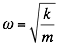# Simple Harmonic Motion of a Spring?

## Homework Statement

If a mass attached to a spring has motion given by the equation X(t) = 5(sin(3pi(t))), what is the equation for the acceleration of the spring? What is the angular speed of the spring,and what is its frequency and period? If the spring has a spring constant of 900 N/m, what is the mass attached at the end of the spring. Assume that the spring is constant, frictionless, and exhibits simple harmonic motion.

## The Attempt at a Solution

I don't even know where to begin on this one. I looked through the slides and nowhere is there a formula for acceleration that doesn't require you to know the mass already. I feel like I'm either missing an equation or missing a variable here that is required to solve this problem. Thanks in advance!

kuruman
Homework Helper
Gold Member
The acceleration is the second derivative of the position. That's all you need for starters.

The acceleration is the second derivative of the position. That's all you need for starters.
Is there a way to do it without taking the derivative? I could do that, but this course is supposed to be solely algebra based...

haruspex
Homework Helper
Gold Member
2020 Award
Is there a way to do it without taking the derivative? I could do that, but this course is supposed to be solely algebra based...
Do you mean it is not supposed to involve calculus?

Do you mean it is not supposed to involve calculus?
Yes. The professor mentioned that calculus is a method of solving these kinds of problems and many others, but he said we are not supposed to use calculus whatsoever.

haruspex
Homework Helper
Gold Member
2020 Award
Yes. The professor mentioned that calculus is a method of solving these kinds of problems and many others, but he said we are not supposed to use calculus whatsoever.
The only alternative I can think of for this problem is to recognise that the equation represents simple harmonic motion and quote the acceleration etc. equations that go with it. That is also suggested by this comment:
Assume that the spring ... exhibits simple harmonic motion.
But calculus is used to obtain those equations, so while you do not write any calculus yourself it is not really avoiding the use of calculus.
Seems a pointless ordinance to me.

The only alternative I can think of for this problem is to recognise that the equation represents simple harmonic motion and quote the acceleration etc. equations that go with it. That is also suggested by this comment:

But calculus is used to obtain those equations, so while you do not write any calculus yourself it is not really avoiding the use of calculus.
Seems a pointless ordinance to me.
I'm glad I'm not the only one who thinks so. Thank you for the advice!

## Homework Statement

If a mass attached to a spring has motion given by the equation X(t) = 5(sin(3pi(t))), what is the equation for the acceleration of the spring? What is the angular speed of the spring,and what is its frequency and period? If the spring has a spring constant of 900 N/m, what is the mass attached at the end of the spring. Assume that the spring is constant, frictionless, and exhibits simple harmonic motion.

## The Attempt at a Solution

I don't even know where to begin on this one. I looked through the slides and nowhere is there a formula for acceleration that doesn't require you to know the mass already. I feel like I'm either missing an equation or missing a variable here that is required to solve this problem. Thanks in advance!, in this case your value of omega is 3pi so you could equate the two and solve for whatever you need.

kuruman
Homework Helper
Gold Member
Yes. The professor mentioned that calculus is a method of solving these kinds of problems and many others, but he said we are not supposed to use calculus whatsoever.
OK then. This problem was not created from a vacuum. Your professor must have taught you something about simple harmonic motion and given you some equations. How about listing the relevant equations? Look at your notes and the textbook.

kuruman
Homework Helper
Gold Member
But calculus is used to obtain those equations, so while you do not write any calculus yourself it is not really avoiding the use of calculus.
Seems a pointless ordinance to me.
Yes, indeed calculus is used to obtain these equations, however the ordinance may not be as pointless as it seems, but designed to test whether one understands the basics of simple harmonic motion.

In simple harmonic motion, the basic idea is that the acceleration is always proportional to the displacement from equilibrium, the constant of proportionality being ##-\omega^2##. One does not need calculus to derive this result for a spring-mass system; it follows from ##F_{net} = ma = -kx## and the definition ##\omega^2 = k/m##. Thus, if one is given an expression for the displacement as a function of time, one can extract ##\omega## from this expression (see post #8) and then just write down the acceleration as a function of time. The rest of the questions may be answered from a knowledge of ##\omega##.

haruspex